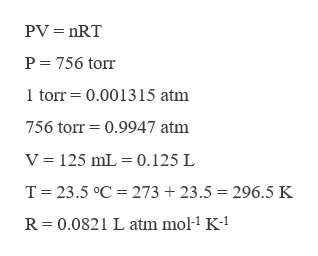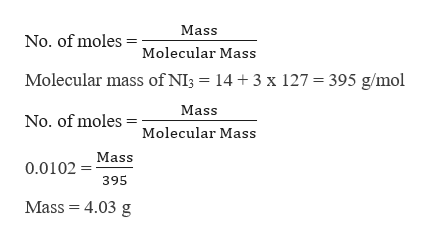# If 125 mL of nitrogen gas, measured at 756 torr and 23.5 oC, react with excess iodine according to the following reaction, which mass of nitrogen triiodide is produced? N2 (g)+ 3I2(s) --> 2NI3(s)

Question

If 125 mL of nitrogen gas, measured at 756 torr and 23.5 oC, react with excess iodine according to the following reaction, which mass of nitrogen triiodide is produced?

N2 (g)+ 3I2(s) --> 2NI3(s)

check_circleExpert Solution
Step 1

To calculate the mass of nitrogen triiodide produced first it is required to calculate the number of moles of nitrogen, which can be done by using the following equation,help_outlineImage TranscriptionclosePV nRT P 756 torr 1 torr 0.001315 atm 756 torr 0.9947 atm V = 125 mL 0.125 L T 23.5 °C 273 23.5 296.5 K R 0.0821 L atm mol-1 K-1 fullscreen
Step 2

Substituting the values to calculate moles of nitrogen,

Step 3

Now as per the balanced chemical reaction, 1 mole of nitrogen forms 2 moles of nitrogen triiodide,

Hence 0.0051 moles will f...help_outlineImage TranscriptioncloseMass No. of moles Molecular Mass Molecular mass of NI3= 14 3 x 127 395 g/mol Mass No. of moles Molecular Mass Mass 0.0102 395 Mass = 4.03 g fullscreen

### Want to see the full answer?

See Solution

#### Want to see this answer and more?

Solutions are written by subject experts who are available 24/7. Questions are typically answered within 1 hour*

See Solution
*Response times may vary by subject and question
Tagged in

### Chemistry